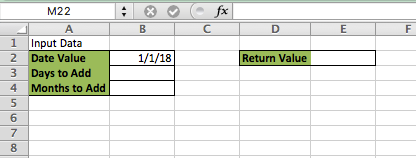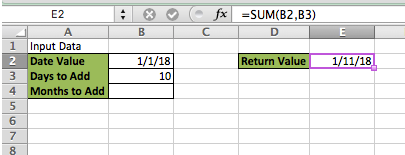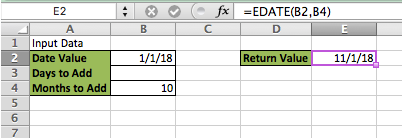Get instant live expert help with Excel or Google Sheets“My Excelchat expert helped me in less than 20 minutes, saving me what would have been 5 hours of work!”

#### Post your problem and you’ll get Expert help in seconds.

Your message must be at least 40 characters
Our professional Expert are available now. Your privacy is guaranteed.

We can quickly add days and months to a date value in Excel. By following a few simple steps, this tutorial will guide any level of Excel in working with dates in Excel

## Setting up the Data

First, we will setup our data so that cell B2 will be the Date Value, cell B3 will be the Days to Add and cell B4 will be the Months to Add. We will then make the return value E2Figure 1 – Setting up the Data

## Adding Days to a Date Value

We can add days to a date value by using a simple SUM formula

SUM Syntax:

`=SUM(Value 1, Value 2)`

• Enter a date in cell B2
• Type “10” in E2
• In cell E2, type this formula: =SUM(B2,10)Figure 2 – SUM Formula for Dates

We can see in this Example, the formula in E2 returns 1/11/18 because we added 10 days to 1/1/18

## Adding Months to a Date Value

The math equation to add months to a Date is Number of Months x Number of Days in Months, but we can’t do this in Excel because all months don’t have the same number of days in them. Therefore, we must account for this variance when creating our formula. We can use the EDATE function for this

EDATE Syntax:

`=EDATE(Starting Date, Number of Months)`

• Delete the days in cell B3
• Type “10” in cell B4
• In cell E2, type this formula =EDATE(B2,B4)Figure 3 – Adding Months to Date using EDATE

We can see in this example that the function automatically calculates the months and returns 11/1/18

## Instant Connection to an Expert through our Excelchat Service:

Most of the time, the problem you will need to solve will be more complex than a simple application of a formula or function. If you want to save hours of research and frustration, try our live Excelchat service! Our Excel Experts are available 24/7 to answer any Excel question you may have. We guarantee a connection within 30 seconds and a customized solution within 20 minutes.

### Did this post not answer your question? Get a solution from connecting with the expert.Another blog reader asked this question today on Excelchat:## Subscribe to Excelchat.coAnother blog reader asked this question today on Excelchat: